# Triangle Solver

Drag the vertices to make the triangle roughly the shape you want.

At least one vertex must be moved before entering the triangle's data.

Click on three labels and enter the size of the angle or side.

Label the vertices     ,         and

When you have entered three attributes click the button below:

## How to Solve a Triangle

In order to find all the attributes of a triangle at least three measurements are needed. In fact only three measurements are needed unless they are the three angles. In that case it is impossible to determine the lengths of the sides and the area as there are an infinite number of similar triangles with thise same three angles.

The following three relationships are used to find the unknown sides and angles.

## Sine Rule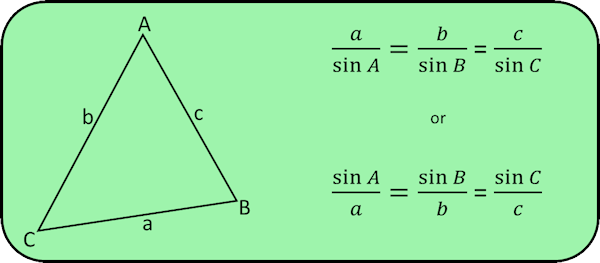## Cosine Rule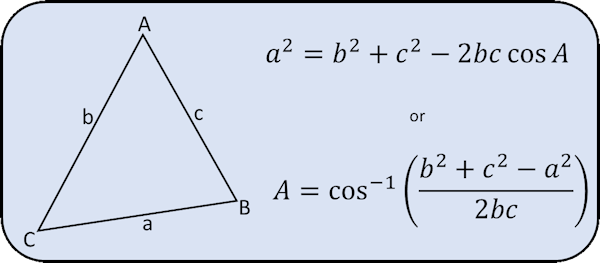## Sine Formula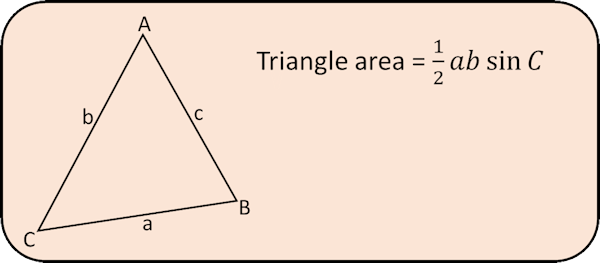## Input

The lengths of sides and size of angles must be all be positive numbers. Each angle must be less than 180o and the longest side must be less than the sum of the shorter two sides.

## Exercises

#### TrigonometryRight-angled triangle trigonometry questions starting with the basics then going on to 3D and exam-style questions.

The short web address is:

Transum.org/go/?to=trig

#### Common Trig Ratios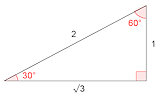A self-marking exercise covering the common trigonometric ratios for certain memorable angles.

The short web address is:

Transum.org/go/?to=commontrigratios

#### Trigonometry Advanced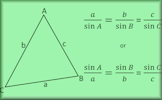A self-marking exercise on the sine rule, cosine rule and the sine formula for finding the area of a triangle.

The short web address is:

Transum.org/go/?to=adtrig

## Comments

Conyers Maths, Twitter

Tuesday, November 29, 2016For All:

©1997-2021 WWW.TRANSUM.ORG

The code for this application was written by Transum using the wonderful functions written by Copyright (c) 2015 Project Nayuki and JS Fiddle.